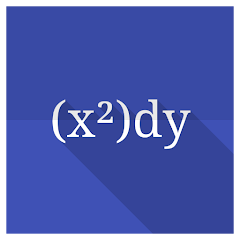# Differential equations

10K+Everyone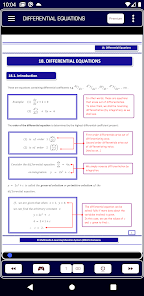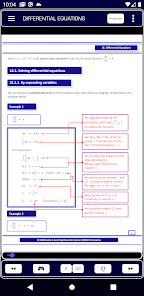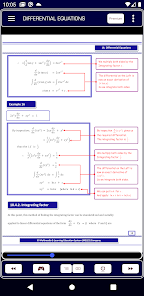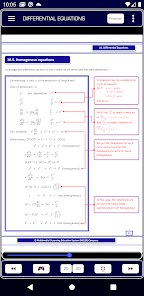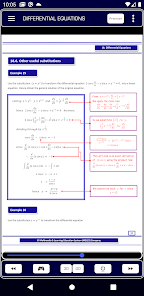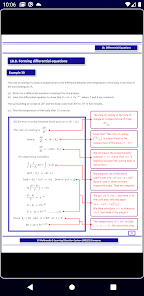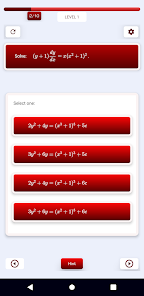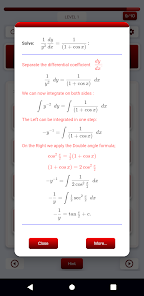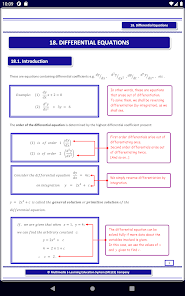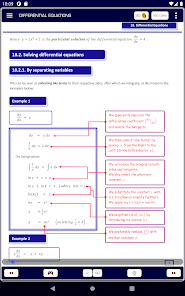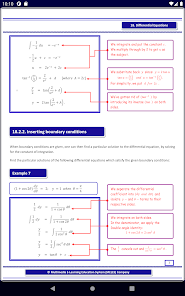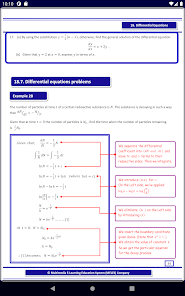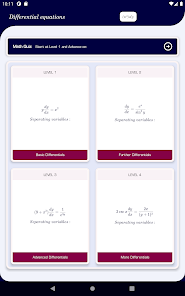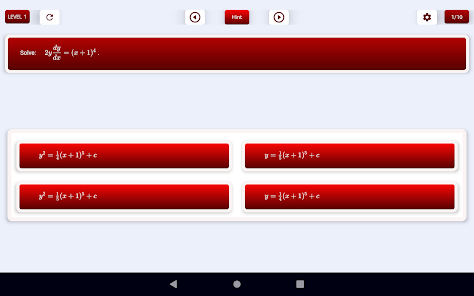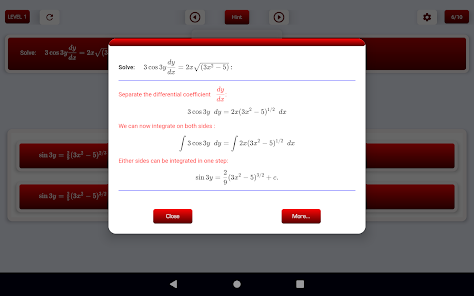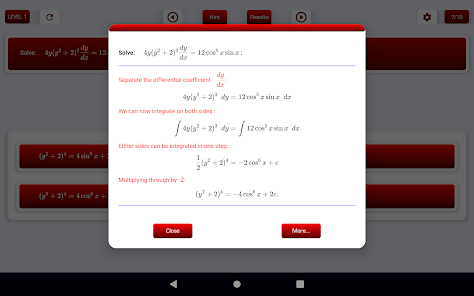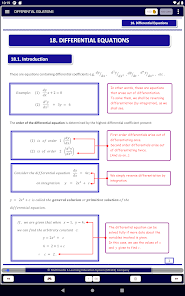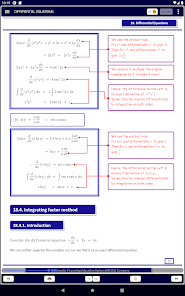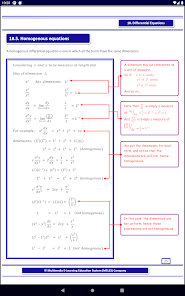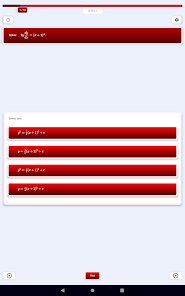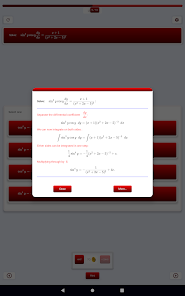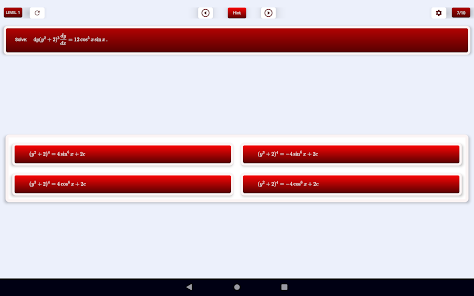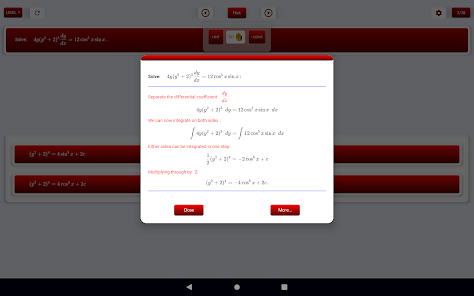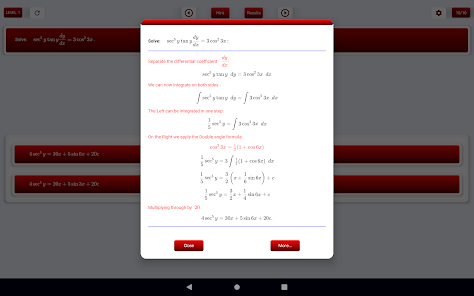Featured sub-topics:
- Solving by separating variables
- Inserting boundary conditions
- Exact differential equations
- Integrating factor method
- Homogeneous equations
- Other useful substitutions
- Differential equation problems
- Forming differential equations

Play the in-app Calculus Quiz to perfect your math skills!

Simplified explanations, plus extra side notes with even more explanation.

Over 30 examples per chapter with step by step working.

Past paper examination questions at the end of each chapter.

Check out other Pure Math Chapters here: